The weblog of Nicholas Chapman Visualising the topology of the rotations with the help of PortalsPosted 10 Oct 2016Summary: I present a way of visualising the topology of the space of rotations (SO(3)), using the analogy of portals from the computer game Portal. I use this analogy to explain Dirac's belt trick in a hopefully intuitive way. The space of possible rotations can be thought of as a sphere, where every point in the sphere defines a rotation in the following way - the rotation is around a vector from the centre of the sphere to the point, in the counter-clockwise direction as usual, with the angle of rotation determined by the length of the vector. This space of rotations is known as the group SO(3) - the group of special orthogonal 3x3 matrices. (Note that in mathematics, rotations themselves don't say anything about paths from an initial to final state. Rather a rotation is more like an 'instantaneous' change in orientation.) The radius of the sphere is $$\pi$$, so the point at the top of the sphere corresponds to a counter-clockwise rotation of $$\pi$$ radians (half a full rotation) around the vertical axis, and the point on the bottom of the sphere corresponds to a counter-clockwise rotation around the down vector. Since this vector is in the opposite direction to the vertical axis, the net result is the same rotation. This means that in the space of rotations these points are (topologically) identical. Likewise, any two points directly opposite each other on the boundary of the sphere are identical. This can be visualised as a a portal connecting any two opposite points, like in the computer game Portal:In the computer game Portal, you can construct portals, a pair of which allow the player or objects to travel ('teleport') from portal to the other. In other words they connect space a bit like a wormhole does in general relativity and science fiction. One portal is always orange, and this portal is connected to the other portal of the pair, which is always blue. Now imagine a path through the space of rotations. Consider a path that goes from the bottom of the sphere to the top. This is a path from $$-\pi$$ to $$\pi$$ rotation around the upwards vertical axis. It is also a loop (closed path), since it ends up back at the same rotation. I like to think of this path as travelling through the portals. This path is shown in the following diagram: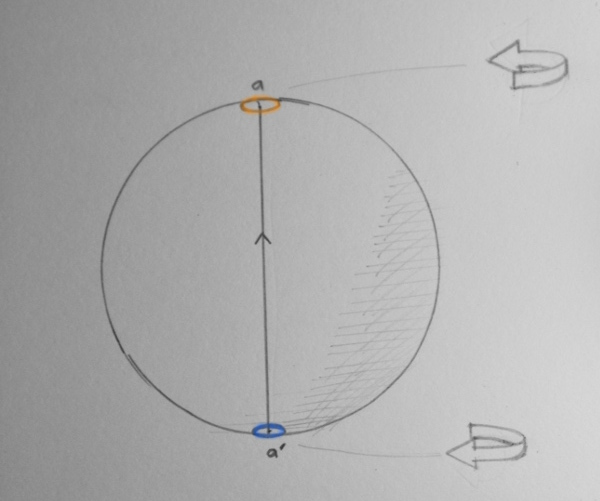A path from $$-\pi$$ to $$\pi$$ rotation We can also illustrate this path with a series of teapots. Each teapot is rotated by an amount given by stepping along the path a certain distance. The left teapot corresponds to the bottom of the path. The middle teapot corresponds to the middle of the path and just has the identity rotation. The right teapot corresponds to the top of the path. Note that the rotations 'wrap around', and it ends up with the same rotation as the left teapot. Now the question arises - can we contract the loop to a single point? e.g. can we continuously deform the loop, so it gets smaller and smaller, until it reaches a single point? It's clear using the portal analogy that this is not possible. If we try and move one of the portals leftwards around the sphere, to make it closer to the other portal, then the other portal moves rightwards, since the portals are constrained to be directly opposite each other! If you think about it, you will see that you can't contract a loop running through the portals to a point. Now let's suppose that we have a path that crosses the space of rotations twice, e.g. a double rotation, or rotation from $$-2\pi$$ to $$2\pi$$.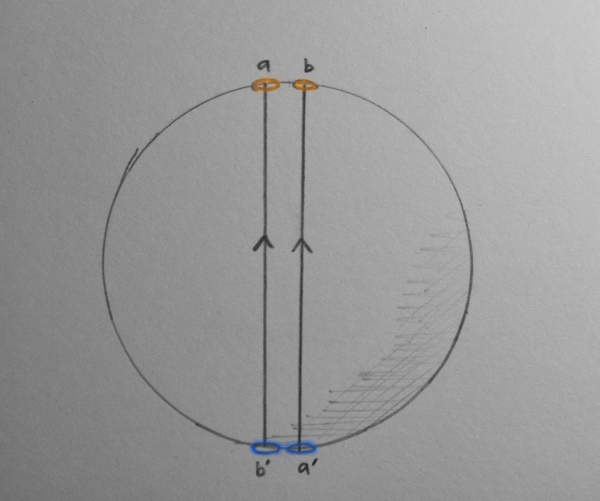A path from $$-2\pi$$ to $$2pi$$ rotation. Note that the path enters portal a and exits portal a', then enters portal b and then exits portal b'. The paths are shown slightly separated for clarity.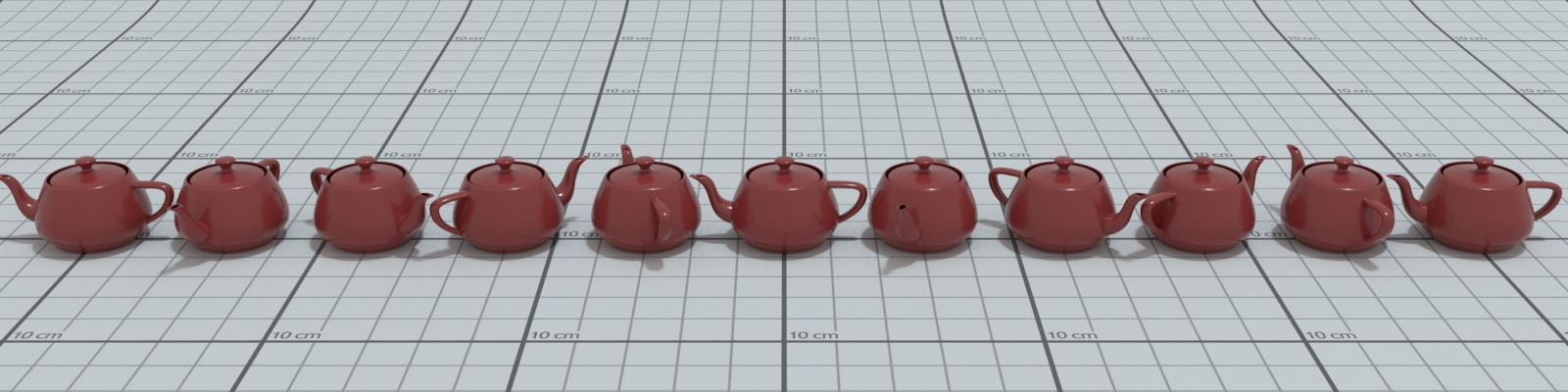A path from $$-2\pi$$ to $$2pi$$ rotation In the double rotation case, we can contract this loop to a single point! To do so, we do the following trick: We rotate one pair of portals continuously around the sphere, until they match up with the other pair. Then the loop disappears to a point!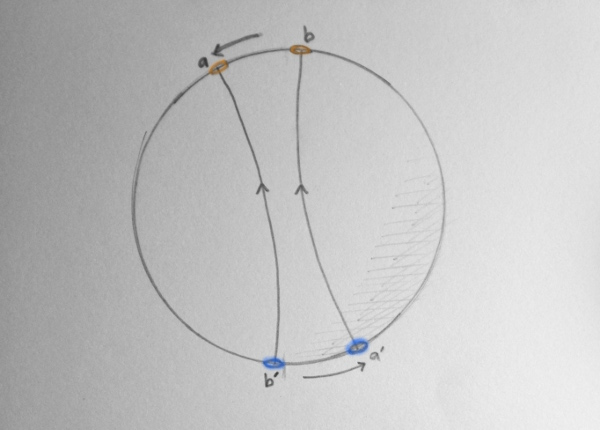A deformation of a $$4\pi$$ rotation.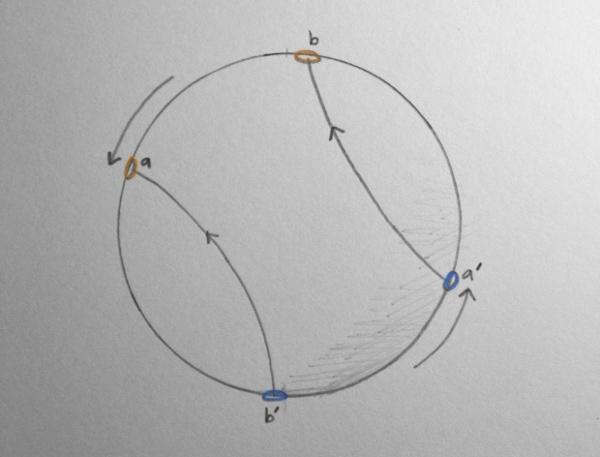A deformation of a $$4\pi$$ rotation.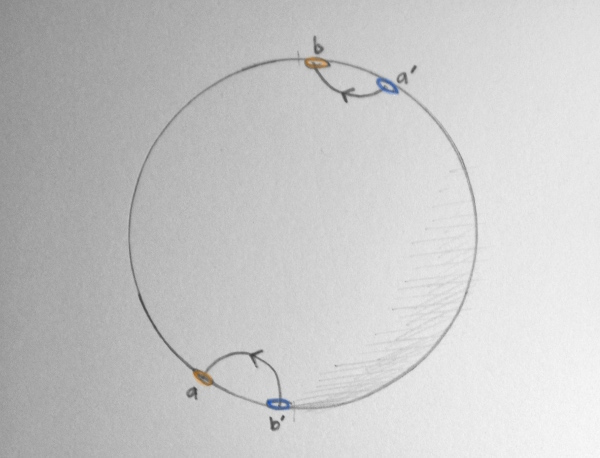A deformation of a $$4\pi$$ rotation.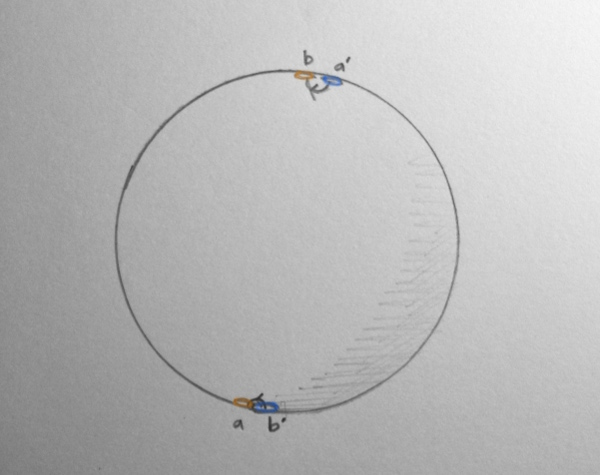A deformation of a $$4\pi$$ rotation. This can be demonstrated in an animation. As time advances in the animation, we are moving the portals around the sphere as above, until the portals meet, and the path has contracted to a single point, at which there is no change in rotation along the path.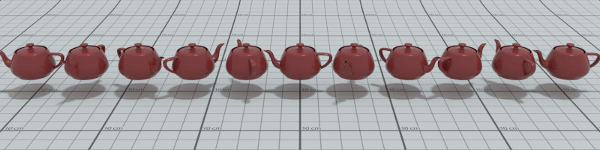Animation of a path through SO(3), starting with $$-2\pi$$ to $$2\pi$$ rotation, deformed continuously until the path vanishes. This continuous deformation of a path from a $$-2\pi$$ to $$2\pi$$ rotation to a point, can be physically demonstrated by twisting a belt, or alternatively with the 'Balinese candle dance', both of which I encourage you to try! (See this video for an example.) Further reading: Comments and corrections welcome! Do you have a comment or feedback about this blog post? Please email me.< Back All content by Nicholas Chapman. Static Pages on this website Our software Indigo Renderer Chaotica Substrata Winter